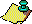Contents Next: The Cubic Iteration Up: Approximations to via the Previous: Iteration Construction

# The Quadratic and Quartic Iterations

In the previous section we showed how to construct a quadratic iteration from the case p=2. In this section we show how the cases p=2 and p=4 relate to the Borwein's quadratic and quartic iterations. For an overview of the method see . For more complete details see , . In this and the next section we summarise the results needed and omit the proofs. Before stating these results, we first give a brief overview.

In the previous section we saw how a quadratic iteration can be constructed using the modular equation (4.6) which relates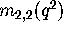and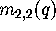. It is useful it introduce auxiliary functions. These functions are modular forms and are usually denoted by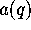,and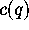. In each case, these auxiliary functions have some remarkable properties:

(1)is some k-th root of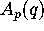(2)
There exist linear relationships between the functions,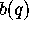,,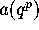,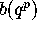and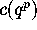.
(3)
There is a simple polynomial equation relating,and. In fact, for the quadratic and quartic iterations of this section and the cubic iteration of the next section this relation is precisely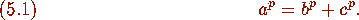Instead of defining a sequence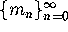which comes from the function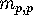we define two auxiliary sequences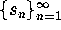and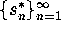which will come from the functions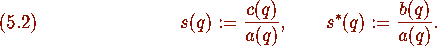In this way, (5.1) becomes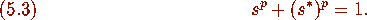It will turn out that the multipliercan be written simply in terms of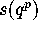.

We now consider the first case p=2. By [4, p.698,] we have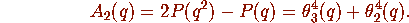Following  we define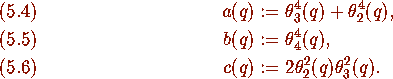Then we have the following results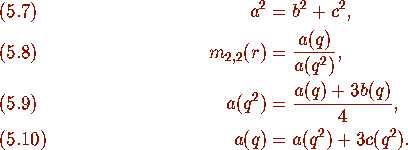Now we let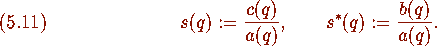Then from (5.7), (5.9)--(5.11) we have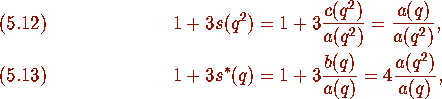and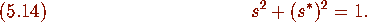Now, by (5.8),(5.12) and (5.13) we have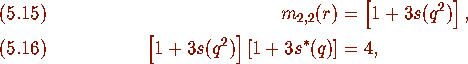and, by (3.18), and (5.15) we have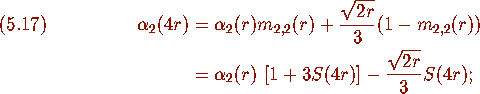where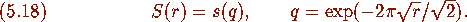Now, from (3.19), (3.20) we know that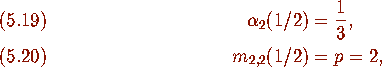and so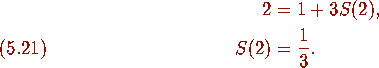By letting,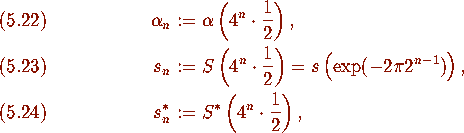we have the following

### Theorem 5.1

(Borwein and Borwein [6, Iteration 3.6, p. 700,]) Define sequences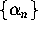,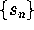, and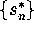by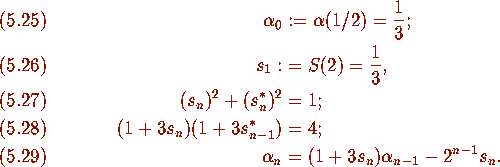Then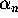converges quadratically to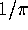.

We now consider the case p=4 and show how it is related to Borwein and Borwein quartic iteration [8, Algorithm1,] and how it coincides with another Borwein and Borwein quartic iteration [6, Iteration 3.4, p. 700,]. From (3.15), [4, Chapter 9,], [6, Theorem 2.2,] we have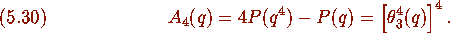Following  we define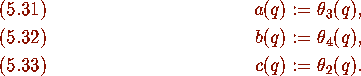Then we have the following results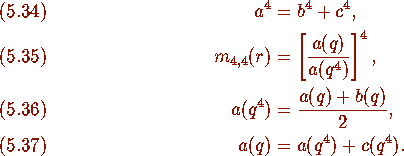As usual we let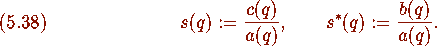Then, by (5.34), (5.36)--(5.37) we have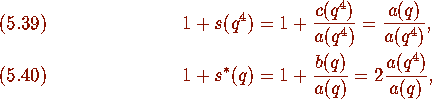and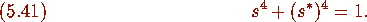Now, from (5.35), (5.39)--(5.41) we have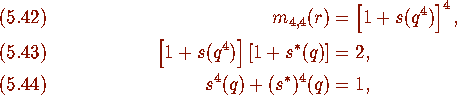and, by (3.18), (5.42) we have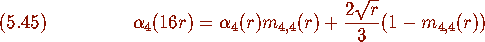where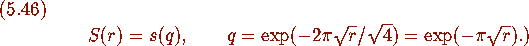From (3.19), (3.20) we know that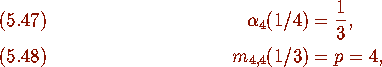and so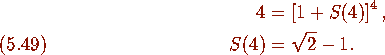By letting,we have the following

### Theorem 5.2

(Borwein and Borwein [6, Iteration 3.4, p. 700,]) Define sequences,, andby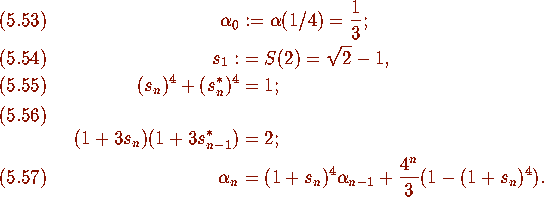Thenconverges quartically to.Contents Next: The Cubic Iteration Up: Approximations to via the Previous: Iteration Construction